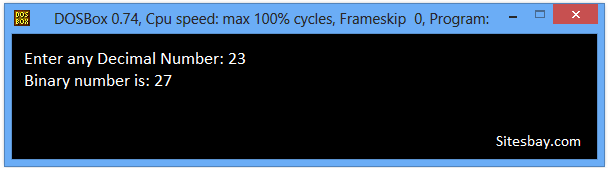# Convert Decimal to Octal Program in C++

## C++ program to Convert Decimal to Octal

A repeated division and remainder algorithm can convert decimal to binary, octal, or hexadecimal.

### Algorithom for Number Conversion

• Divide the decimal number by the desired target radix (2, 8, or 16).
• Append the remainder as the next most significant digit.
• Repeat until the decimal number has reached zero.

Before write decimal to octal conversion code you must be basic kanowledge about For Loop in C++, While Loop in C++ and Modulus Operator in C++.

## C++ program to convert Decimal to Octal

```
#include<iostream.h>
#include<conio.h>

void main()
{
int no,rem,i=0,j;
clrscr();
cout<<"Enter any Decimal Number: ";
cin>>no;
while(no>0)
{
rem[i]=no%8;
i++;
no=no/8;
}
cout<<"Binary Number is: ";
for(j = i - 1; j > = 0 ; j--)
{
cout<<rem[j];
}
getch();
}
```

## Output

```Enter any Decimal Number: 23
Binary number is: 27
```## Pure VPN Privide Lowest Price VPN Just @ \$1.65. Per Month with Non Detected IP Lowest Price Non Detected IP VPN